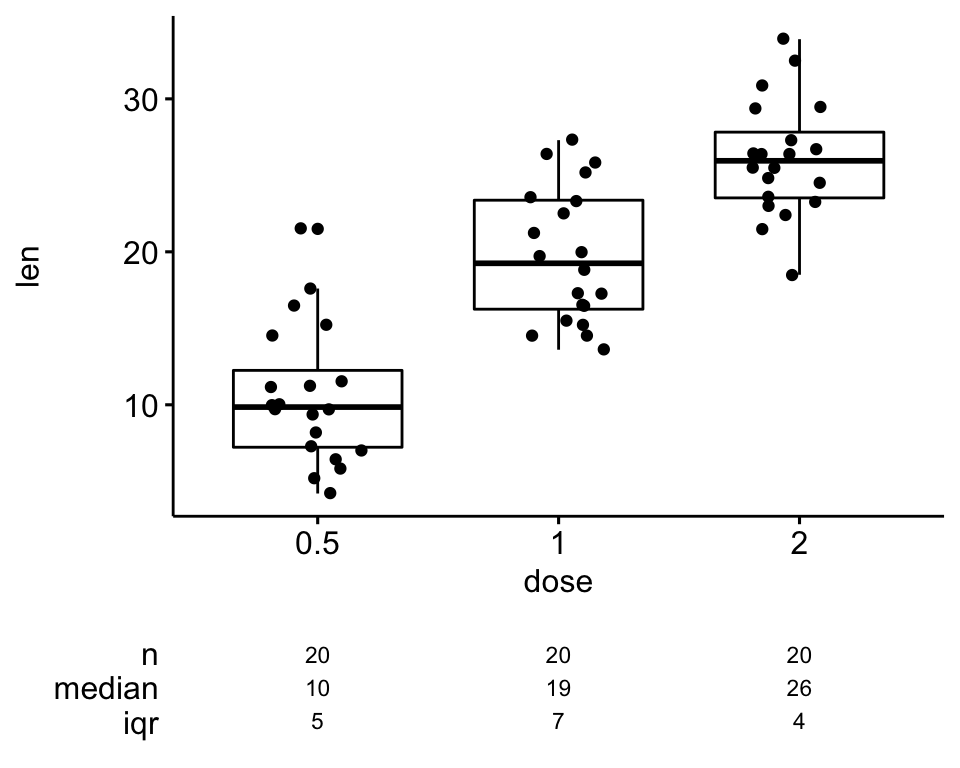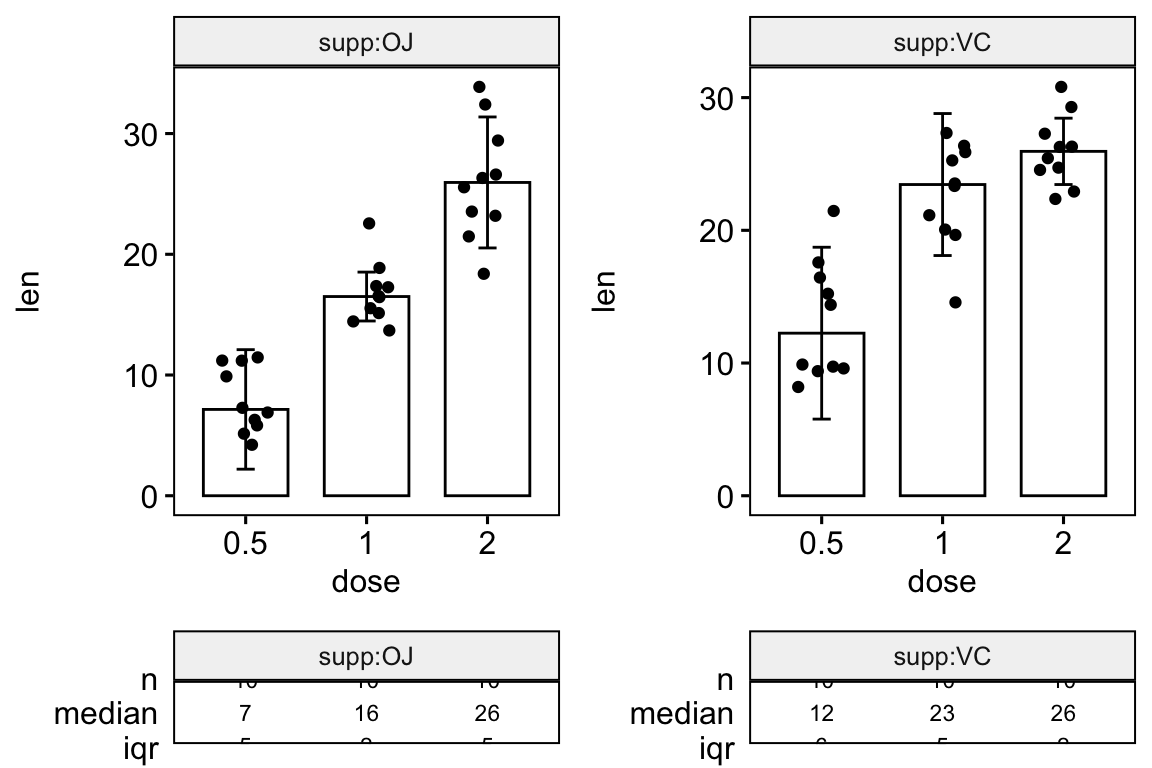# How to Create a Beautiful Plots in R with Summary Statistics Labels#### How to Create a Beautiful Plots in R with Summary Statistics Labels

You will learn how to create beautiful plots in R and add summary summary statistics table such as sample size (n), median, mean and IQR onto the plot. We will also describes how to create multipanel graphics combined with the summary table. Examples of plots illustrated here, include: box plot, violin plot, bar plot, line plot; etc.

Contents:

## Prerequisites

library(tidyverse)
library(rstatix)
library(ggpubr)

Data preparation:

# Demo data
data("ToothGrowth")
df <- ToothGrowth
df$dose <- as.factor(df$dose)
set.seed(123)
qc <- rep(c("pass", "fail"), 30)
dfqc <- as.factor(sample(qc, 60)) # Inspect the data head(df) ## len supp dose qc ## 1 4.2 VC 0.5 fail ## 2 11.5 VC 0.5 pass ## 3 7.3 VC 0.5 fail ## 4 5.8 VC 0.5 pass ## 5 6.4 VC 0.5 pass ## 6 10.0 VC 0.5 pass ## Basic box plots with add summary statistics In the following R code, possible values for the argument ggfunc are the ggpubr R package functions, including: ggboxplot, ggviolin, ggdotplot, ggbarplot, ggline, etc. It can be also any other ggplot function that accepts the following arguments: data, x, color, fill, palette, ggtheme, facet.by. # Basic plot ggsummarystats( df, x = "dose", y = "len", ggfunc = ggboxplot, add = "jitter" )# Color by groups ggsummarystats( df, x = "dose", y = "len", ggfunc = ggboxplot, add = "jitter", color = "dose", palette = "npg" )Note that, you can create step by step your own graph and summary table. The following R code describes how to combine the main graph and the summary table into one figure. # Compute summary statistics summary.stats <- df %>% group_by(dose) %>% get_summary_stats() %>% select(dose, n, median, iqr) summary.stats # Create a boxplot bxp <- ggboxplot( df, x = "dose", y = "len", add = "jitter", ggtheme = theme_bw() ) # Visualize the summary statistics summary.plot <- ggsummarytable( summary.stats, x = "dose", y = c("n", "median", "iqr"), ggtheme = theme_bw() ) + clean_table_theme() # Combine the boxplot and the summary statistics plot ggarrange( bxp, summary.plot, ncol = 1, align = "v", heights = c(0.80, 0.20) ) ## Grouped plots with summary table ### Grouped box plots and violin plots # Grouped plots ggsummarystats( df, x = "dose", y = "len", ggfunc = ggboxplot, add = "jitter", color = "supp", palette = "npg" ) # Change plot type to violin ggsummarystats( df, x = "dose", y = "len", ggfunc = ggviolin, add = c("jitter", "median_iqr"), color = "supp", palette = "npg" )### Grouped bar plots and line plots # Create barplot ggsummarystats( df, x = "dose", y = "len", ggfunc = ggbarplot, add = c("jitter", "median_iqr"), position = position_dodge(), color = "supp", palette = "npg" ) # Create line plots ggsummarystats( df, x = "dose", y = "len", ggfunc = ggline, add = c("jitter", "median_iqr"), color = "supp", palette = "npg" )### Three groups on the x axis ggsummarystats( df, x = "supp", y = "len", ggfunc = ggboxplot, add = c("jitter"), color = "dose", palette = "npg" )## Multipanel plots with summary table Key arguments: • facet.by: character vector, of length 1 or 2, specifying grouping variables for faceting the plot into multiple panels. Should be in the data. • labeller: Character vector. Possible values are one of label_both (panel labelled by both grouping variable names and levels) and label_value (panel labelled with only grouping levels). Create panels according to one grouping variable: ggsummarystats( df, x = "dose", y = "len", ggfunc = ggboxplot, add = c("jitter"), color = "dose", palette = "jco", facet.by = "supp", labeller = "label_value", ggtheme = theme_bw(), legend = "top" )Create panels according to two grouping variables ggsummarystats( df, x = "dose", y = "len", ggfunc = ggboxplot, add = c("jitter"), color = "dose", palette = "jco", facet.by = c("supp", "qc"), labeller = "label_both", ggtheme = theme_bw(), legend = "top" )Create independent panels using the argument free: ggsummarystats( df, x = "dose", y = "len", ggfunc = ggboxplot, add = c("jitter"), color = "dose", palette = "jco", facet.by = c("supp", "qc"), labeller = "label_both", free.panels = TRUE, ggtheme = theme_bw(), legend = "top" )## Build step by step a custom multipanel plot Create a multipanel box plot using one grouping variable (supp): # Group the data by supp # Apply the function ggsummarystats to each subset df.grouped <- df %>% df_split_by(supp, label_col = "panel", labeller = df_label_both) %>% mutate(plot_list = map( data, ggsummarystats, x = "dose", y = "len", ggfunc = ggbarplot, add = c("jitter", "median_iqr"), facet.by = "panel" ) ) df.grouped ## # A tibble: 2 x 4 ## supp data panel plot_list ## <fct> <list> <fct> <list> ## 1 VC <tibble [30 × 4]> supp:OJ <ggsmmrys> ## 2 OJ <tibble [30 × 4]> supp:VC <ggsmmrys> # Print the plots plot_list <- df.groupedplot_list
class(plot_list) <- c("ggsummarystats_list", "list")
print(plot_list)•Jegor

I the function ggsummarystats in the package that is available on CRAN? Because I dont find it.

•Kassambara

The ggsummarystats() is available in the ggpubr dev version. You can install it as follow:

if(!require(devtools)) install.packages("devtools")
devtools::install_github("kassambara/ggpubr")

A CRAN released is planned for next week.

•Anh Luong

Hi, I keep getting all this warnings nonstop:
Warning message in stats::qt(alpha/2, .data$n – 1): “NaNs produced” Warning message in stats::qt(alpha/2, .data$n – 1):
“NaNs produced”
Warning message in stats::qt(alpha/2, .data$n – 1): “NaNs produced” Warning message in stats::qt(alpha/2, .data$n – 1):
“NaNs produced”
Warning message in stats::qt(alpha/2, .data\$n – 1):
“NaNs produced”

••